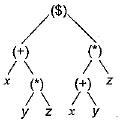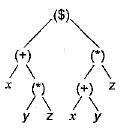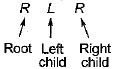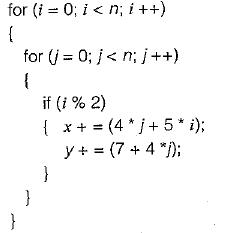Courses

# Code Generation And Optimization (Basic Level) - 1

## 10 Questions MCQ Test Question Bank for GATE Computer Science Engineering | Code Generation And Optimization (Basic Level) - 1

Description
This mock test of Code Generation And Optimization (Basic Level) - 1 for Computer Science Engineering (CSE) helps you for every Computer Science Engineering (CSE) entrance exam. This contains 10 Multiple Choice Questions for Computer Science Engineering (CSE) Code Generation And Optimization (Basic Level) - 1 (mcq) to study with solutions a complete question bank. The solved questions answers in this Code Generation And Optimization (Basic Level) - 1 quiz give you a good mix of easy questions and tough questions. Computer Science Engineering (CSE) students definitely take this Code Generation And Optimization (Basic Level) - 1 exercise for a better result in the exam. You can find other Code Generation And Optimization (Basic Level) - 1 extra questions, long questions & short questions for Computer Science Engineering (CSE) on EduRev as well by searching above.
QUESTION: 1

### A non relocatable program is one which

Solution:

The phases of compiler
1. Lexical
2. Syntax
3. Semantic
4. Intermediate code generation, are all machine dependent phases and are not relocatable.
The together make pass-1 of the compiler. This program hence can not be made to execute in any area of storage other than the one designated for it at the time of its coding or translation.

QUESTION: 2

### A self relocating program is one which

Solution:

The self relocating program is one which can itself perform the relocation of its address sensitive portion. Code-optimization and target code generation comes under the pass-2 of the compiler and is machine independent.

QUESTION: 3

### Generation of intermediate code based on a abstract machine model is useful in compilers because

Solution:

By the help of intermediate code generation, the portability of the front end of the compiler enhances.

QUESTION: 4\$ denotes exponentiation. If the tree is traversed in preorder, which, if any, of the expressions below is formed?

Solution:The preorder expansion can be represented as\$ + x*yz* + xyz

QUESTION: 5

Which of the following cannot be used as an intermediate code form?

Solution:

Quadruples is not the intermediate code form.

QUESTION: 6

A basic block can be analyzed by

Solution:

Properties of a basic block are as follows:

• The flow of control can only enter the basic block through the first instruction in the block.
• No numps in the middle of the block.
• Control leaves the block without halting/branching.

The basic blocks become the nodes of a flow graph, whose edges indicate which blocks can follows which other blocks.

QUESTION: 7

The graph that shows the basic blocks and their successor relationship is called

Solution:

The graph that shows the basic blocks and their successor relationship is called control graph.

QUESTION: 8

Consider the following C code segment:Which one of the following is false?

Solution:

(a) i%2 is inner loop invariant, it can be moved before inner loop.
(b) 4*j is common sub-expression-appeared in two statements.
(c) 4*j can be reduced to j<<2 by strength reduction.
(d) There is no dead code in given code segment. So there is no scope of dead code elimination in this code.
Hence only option (d) is FALSE.

QUESTION: 9

Some code optimizations are carried out on the intermediate code because

Solution:

Some code optimizations are carried out on the intermediate code because program analysis is more accurate on intermediate code than on machine code.

QUESTION: 10

Which languages necessarily need heap allocation in the runtime environment?

Solution:

Runtime environment means we deal with dynamic memory allocation and Heap is a dynamic data structure.
So it is clear that those languages that allow dynamic data structure necessarily need heap allocation in the runtime environment.